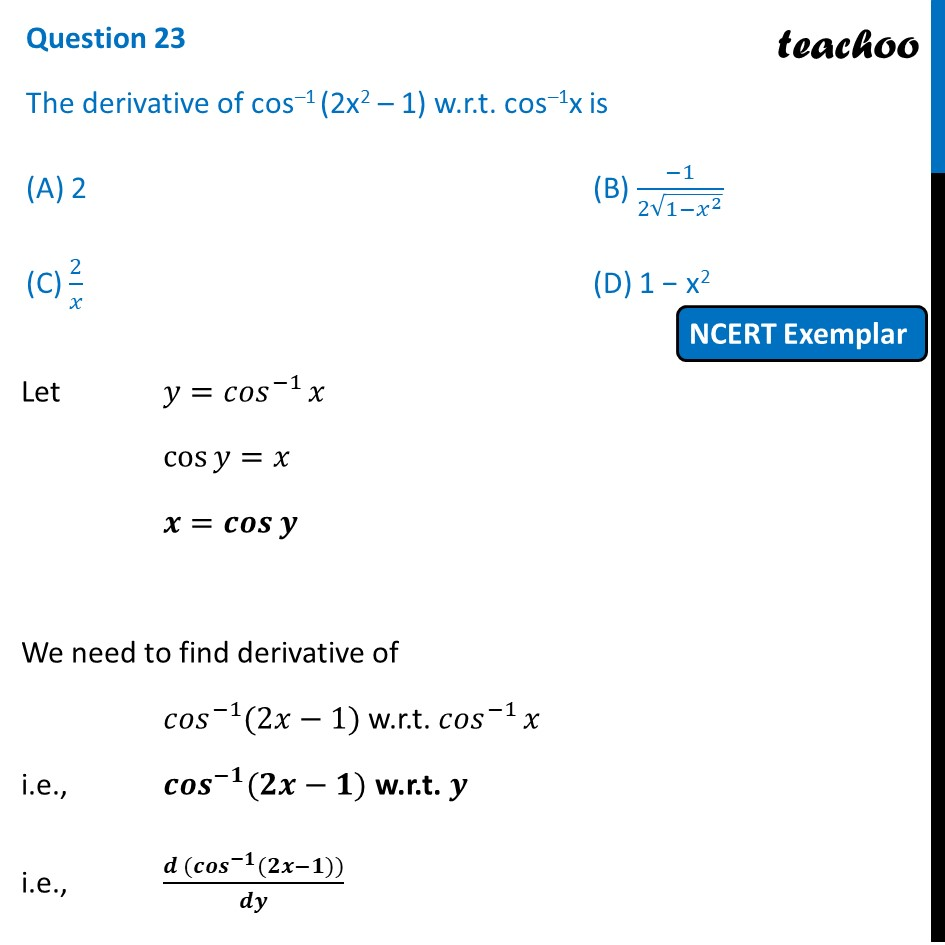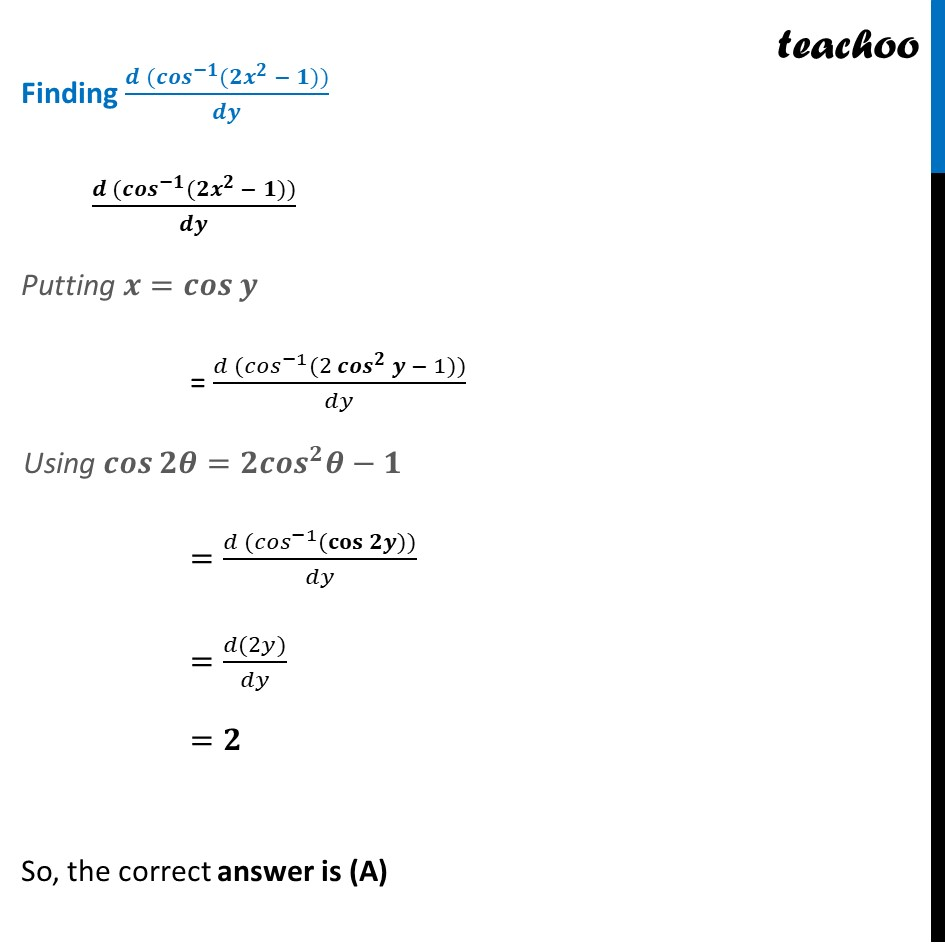NCERT Exemplar - MCQs

Chapter 5 Class 12 Continuity and Differentiability
Serial order wise

## (D) 1 − x 2

This question is similar to Question 24 - CBSE Class 12 - Sample Paper for 2022 BoardsLearn in your speed, with individual attention - Teachoo Maths 1-on-1 Class

### Transcript

Question 21 The derivative of cos–1 (2x2 – 1) w.r.t. cos–1x is (A) 2 (B) (−1)/(2√(1−𝑥^2 )) (C) 2/𝑥 (D) 1 − x2 Let 𝑦=〖𝑐𝑜𝑠〗^(−1)⁡𝑥 cos⁡〖𝑦=𝑥〗 〖𝒙=𝒄𝒐𝒔〗⁡𝒚 We need to find derivative of 〖𝑐𝑜𝑠〗^(−1)⁡〖(2𝑥−1)〗 w.r.t. 〖𝑐𝑜𝑠〗^(−1)⁡𝑥 i.e., 〖𝒄𝒐𝒔〗^(−𝟏)⁡〖(𝟐𝒙−𝟏)〗 w.r.t. 𝒚 i.e., (𝒅 〖(〖𝒄𝒐𝒔〗^(−𝟏)〗⁡〖(𝟐𝒙−𝟏)〗))/𝒅𝒚 Finding (𝒅 〖(〖𝒄𝒐𝒔〗^(−𝟏)〗⁡〖(𝟐𝒙^𝟐 − 𝟏)〗))/𝒅𝒚 (𝒅 〖(〖𝒄𝒐𝒔〗^(−𝟏)〗⁡〖(𝟐𝒙^𝟐 − 𝟏)〗))/𝒅𝒚 Putting 〖𝒙=𝒄𝒐𝒔〗⁡𝒚 = (𝑑 〖(〖𝑐𝑜𝑠〗^(−1)〗⁡〖(2 〖𝒄𝒐𝒔〗^𝟐⁡𝒚 − 1)〗))/𝑑𝑦 Using 𝒄𝒐𝒔⁡〖𝟐𝜽=𝟐〖𝒄𝒐𝒔〗^𝟐 𝜽−𝟏〗 =(𝑑 〖(〖𝑐𝑜𝑠〗^(−1)〗⁡〖(𝐜𝐨𝐬⁡𝟐𝒚)〗))/𝑑𝑦 =(𝑑(2𝑦))/𝑑𝑦 =𝟐 So, the correct answer is (A)